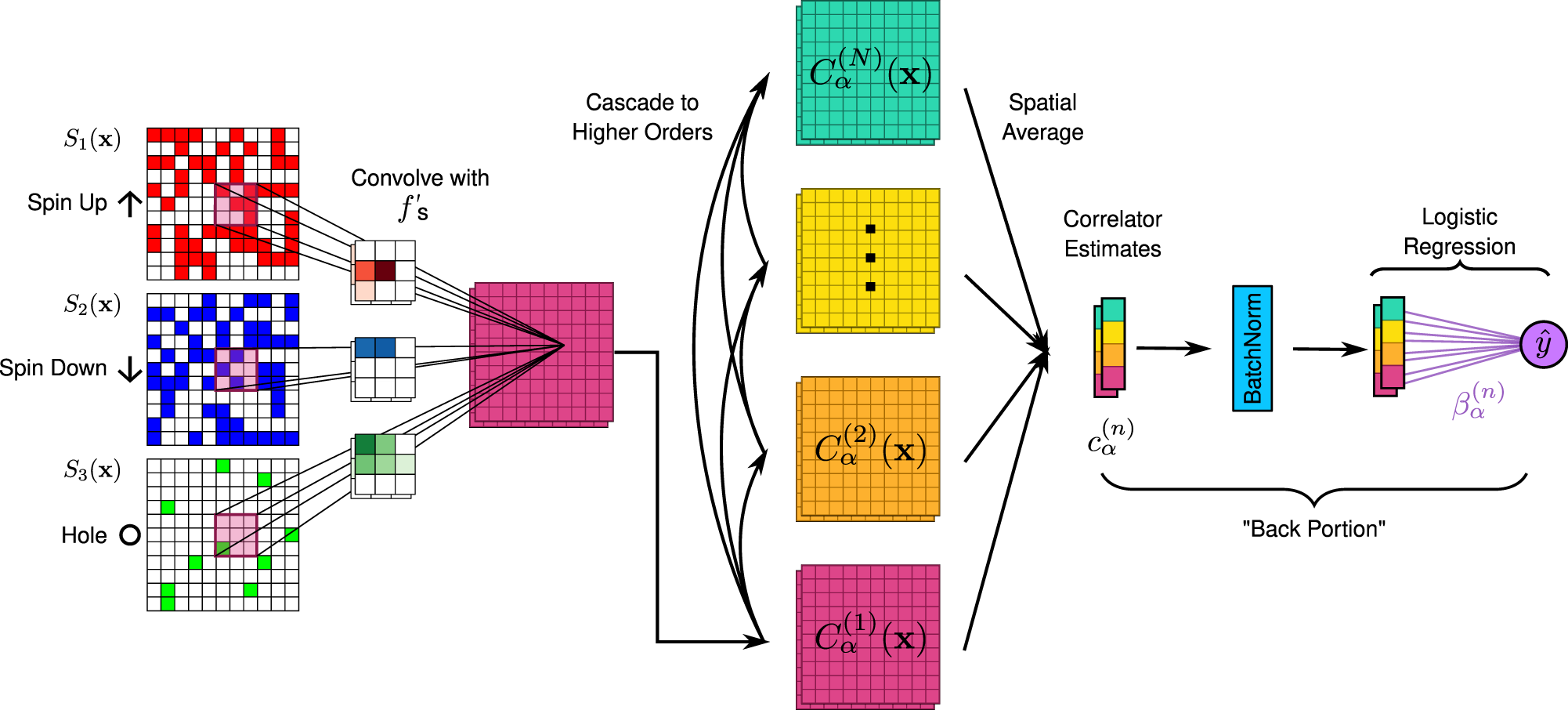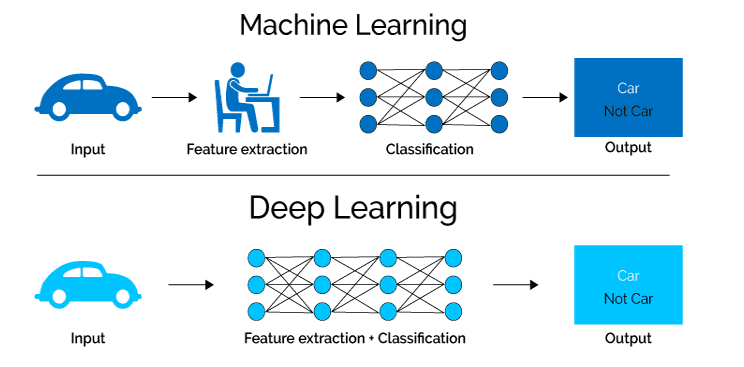# Convolutional Neural Network

The Best Web Development Agency in UAEAccording to Wikipedia, the path for analyzing visual imagery is made possible with the use of deep learning. This act requires the use of a convolutional neural network (CNN, or ConvNet) from a class of artificial neural networks (ANN).

CNNs, as they are fondly called by most, are also known as shift-invariant or space-invariant artificial neural networks (SIANN), based on the shared-weight architecture of the convolution kernels or filters that slide along input features and provide translation-equivariant responses known as feature maps.

This post will give a better understanding of what a convolutional neural network is, its features, uses, importance, and drawbacks. At the end of this read, you will have a new understanding of deep learning, and likewise more insights into Neural Networks.

### What is a convolutional neural network?

A convolutional neural network (CNN) is a type of deep learning neural network that is designed to process data that has a grid-like topology, such as an image.

CNNs are composed of multiple layers, including an input layer, hidden layers, and an output layer. The hidden layers of a CNN typically consist of multiple layers of convolutional and pooling layers, which are designed to extract features from the input data and reduce its dimensionality.

These features are then passed to the output layer, which uses them to make predictions or classifications. CNNs are particularly useful for image and video recognition tasks and are widely used in computer vision applications.### What is a convolutional neural network in deep learning?

In deep learning, a convolutional neural network (CNN) is a type of neural network architecture that is particularly well-suited for image and video recognition tasks. CNNs are composed of multiple layers, including an input layer, hidden layers, and an output layer. The hidden layers of a CNN typically consist of multiple layers of convolutional and pooling layers, which are designed to extract features from the input data and reduce its dimensionality.

### How does a convolutional neural network work?

The convolutional layers in a CNN apply a set of filters to the input data, which are used to identify specific features in the image. These features are then passed through a series of pooling layers, which reduce the spatial resolution of the data, making the network more robust to small translations and deformations in the input.

The output of the pooling layers is then passed through one or more fully connected layers, which use the extracted features to make predictions or classifications. The final output of the CNN is a probability map or a class label, indicating the probability of the input image belonging to a particular class.

CNNs are widely used in computer vision applications such as image classification, object detection, and semantic segmentation.### Why is it called convolutional?

CNN is called "convolutional" because it uses a mathematical operation called "convolution" in the hidden layers of the network.

Convolution is a mathematical operation that combines two functions (in this case, the input image and a set of filters) to produce a third function (the output feature map). The convolution operation is performed by sliding the filters over the input image, element-wise multiplying the values at each position, and summing them up. This operation is done in such a way that the output feature map will have smaller dimensions than the input image; it is called down-sampling or spatial-variant filtering.

The convolution operation is applied multiple times by stacking multiple convolutional layers, each one with multiple filters, which allows the network to extract different features of the image at different levels of abstraction.

This process of convolution and down-sampling helps CNNs automatically and adaptively learn spatial hierarchies of features from input images, making it possible to extract useful features while reducing the dimensionality of the data. This is why it is called a convolutional neural network.

### Conclusion.

Convolutional neural networks are specialized varieties of artificial neural networks that, in at least one of their layers, substitute the mathematical operation known as "convolution" for general matrix multiplication. They are employed in image processing and recognition since they were created primarily to process pixel data.

Jan 24, 2023     by Oluwafemi Smith     161 Views     0FacebookTwitterLinkedinWhatsAppPinterest